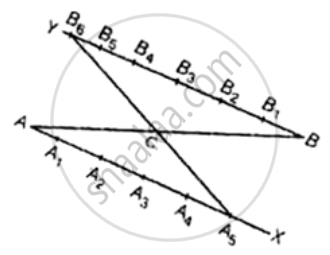# To divide a line segment AB in the ratio 5 : 6, draw a ray AX such that ∠BAX is an acute angle, then draw a ray BY parallel to AX and the points A1, A2 - Mathematics

MCQ
Fill in the Blanks

To divide a line segment AB in the ratio 5 : 6, draw a ray AX such that ∠BAX is an acute angle, then draw a ray BY parallel to AX and the points A1, A2, A3, ....... and B1, B2, B3,....... are located at equal distances on ray AX and BY, respectively. Then the points joined are ______.

• A5 and B6

• A6 and B5

• A4 and B5

• A5 and B4

#### Solution

To divide a line segment AB in the ratio 5 : 6, draw a ray AX such that ∠BAX is an acute angle, then draw a ray BY parallel to AX and the points A1, A2, A3, …….. and B1, B2, B3,………. are located at equal distances on ray AX and B4, respectively. Then the points joined are A5 and B6.

Explanation:-

To divide line segment AB in the ratio 5:6.Steps of construction:-

1. Draw a ray AX making an acute ∠BAX.

2. Draw a ray BY parallel to AX by taking ∠ABY equal to ∠BAX.

3. Divide AX into five (m = 5) equal parts AA1, A1A2, A2A3, A3Aand A4A5

4. Divide BY into six (n = 6) equal parts and BB1, B1B2, B2B3, B3B4, B4B5 and B5B6.

5. Join BA5. Let it intersect AB at a point C. Then, AC : BC = 5 : 6

Concept: Division of a Line Segment
Is there an error in this question or solution?

#### APPEARS IN

NCERT Mathematics Exemplar Class 10
Chapter 10 Construction
Exercise 10.1 | Q 3 | Page 114
Share